## Differential Amplifier with two Op Amps:

The gain as well as input resistance of the differential amplifier can be increased by using two op-amps. The characteristics of such an amplifier are identical to those of a noninverting amplifier. A practical circuit using differential amplifier with two op amp is given in Fig. 34.46.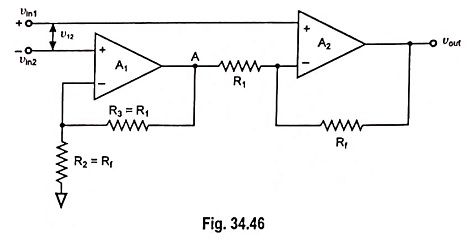The circuit is composed of a two stage amplifier, the first stage being of a noninverting amplifier and the second being of a differential amplifier, both having gains of different values.

### Equations of First Stage and Second Stage:

The output of first stage,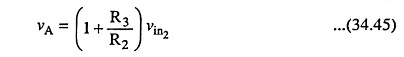Output from second stage,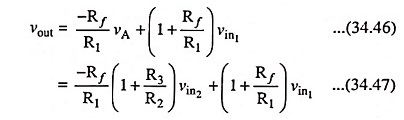Since R3 = R1 and Rf = R2, we have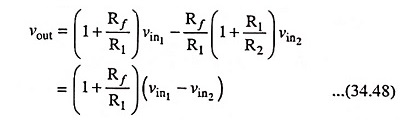Voltage gain,Input Resistance: Input resistance of first stage,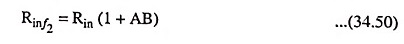where Rin is the open-loop resistance of op-amp, as first stage is noninverting amplifier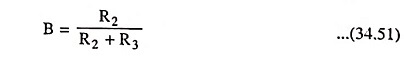With vin2 grounded i.e. vin2 = 0, the second stage also becomes a noninverting amplifier whose input resistance may be given as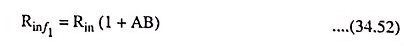where Rin is open-loop resistance of op-amp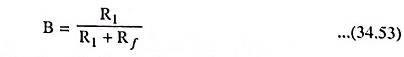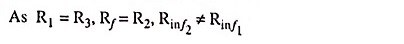### Drawbacks:

The output signal may be of smaller magnitude than expected. This is a drawback of such an amplifier.

Scroll to Top